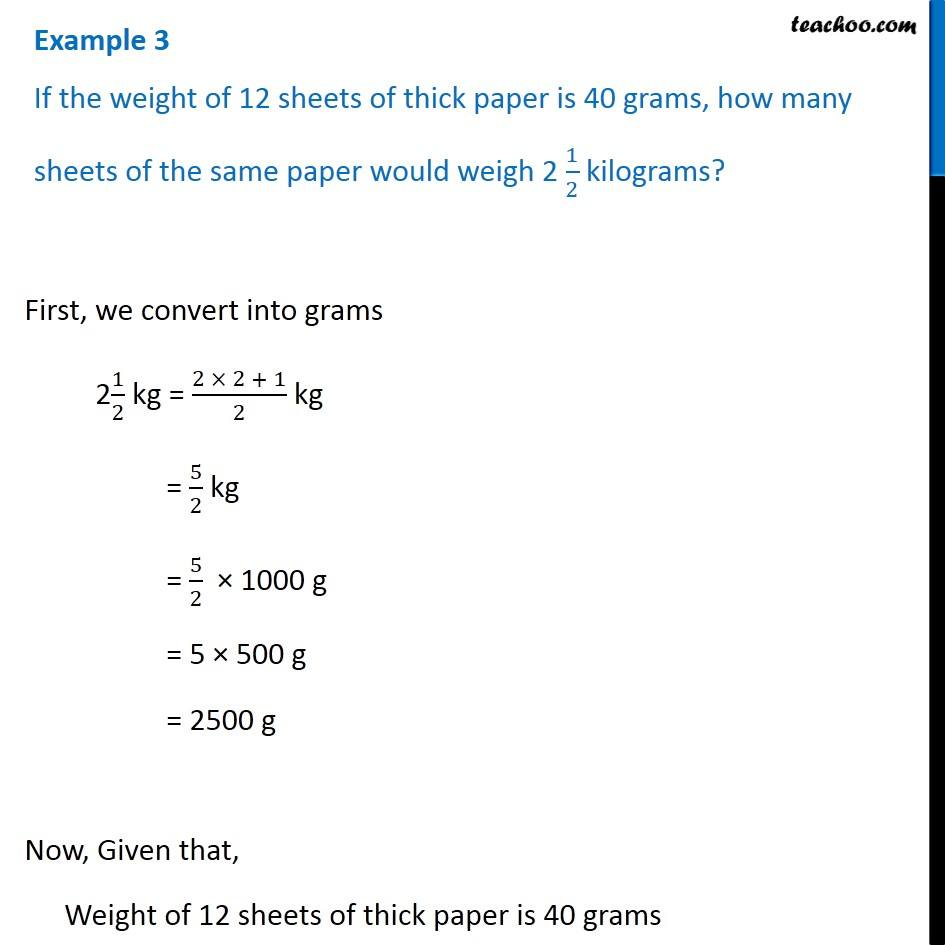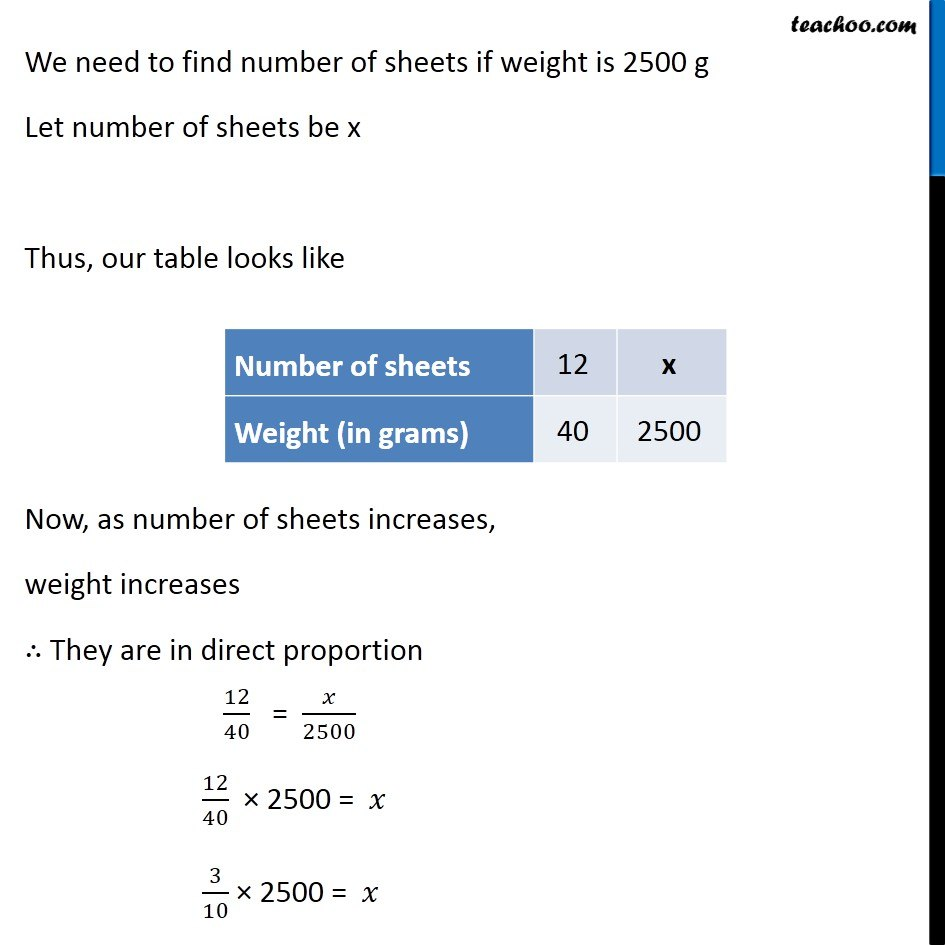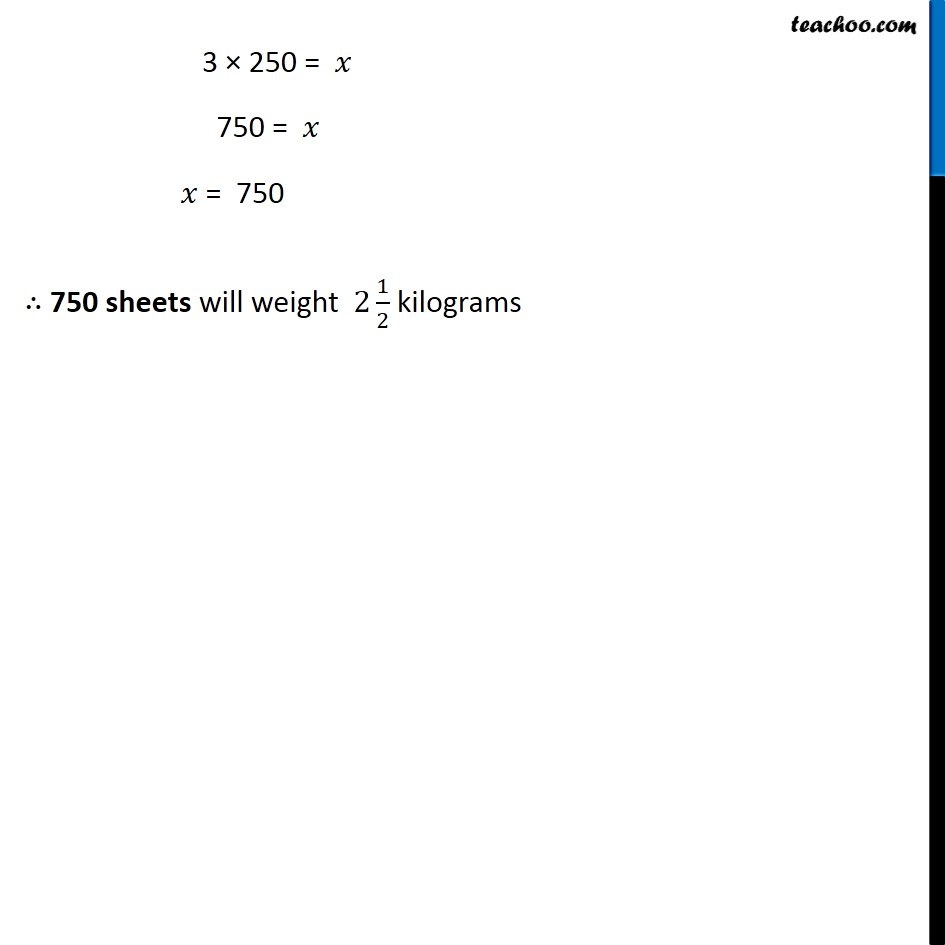Examples

Chapter 11 Class 8 Direct and Inverse Proportions
Serial order wiseLearn in your speed, with individual attention - Teachoo Maths 1-on-1 Class

### Transcript

Example 3 If the weight of 12 sheets of thick paper is 40 grams, how many sheets of the same paper would weigh 2 1/2 kilograms? First, we convert into grams 21/2 kg = (2 × 2 + 1)/2 kg = 5/2 kg = 5/2 × 1000 g = 5 × 500 g = 2500 g Now, Given that, Weight of 12 sheets of thick paper is 40 grams We need to find number of sheets if weight is 2500 g Let number of sheets be x Thus, our table looks like Now, as number of sheets increases, weight increases ∴ They are in direct proportion 12/40 = 𝑥/2500 12/40 × 2500 = 𝑥 3/10 × 2500 = 𝑥 3 × 250 = 𝑥 750 = 𝑥 𝑥 = 750 ∴ 750 sheets will weight 2 1/2 kilograms